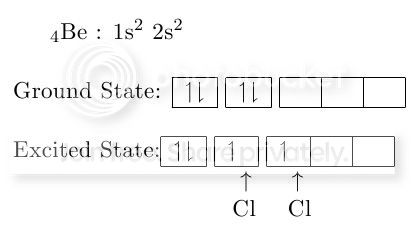# Drawing Benzene resonance mechanism, and reaction mechanism + curly arrows

I am new to LaTeX and there are few things at which I am stuck.

I can draw benzene with alternate double bonds but I don't know how to draw curly arrows to show bond shifting and electrophile attack.I would like to show reaction mechanism of benzene like this:

and

EDIT

There is one another thing I need an expert opinion. I wanted to show covalent bonding in comparison with excited and grounded state of electron. This is the code that I have written. Is there any better way to show Cl sharing its electron than what I have done (adding lots of space in my opinion is not a best way)?

And is possible to draw a curly arrow from the second box to the third to show that an electron is given energy to go to a higher energy level?\documentclass [11pt] {book}
\usepackage[utf8x]{inputenc}
\usepackage{ucs}
\usepackage{amsmath}
\usepackage{amsfonts}
\usepackage{amssymb}
\usepackage{chemfig}
\usepackage{tikz}
\begin{document}
$_4$Be~: 1s$^2$~2s$^2$
\\
\\
Ground State:
\begin{tabular}{|c|}
\hline $\upharpoonleft$$\downharpoonright \\ \hline \end{tabular} \begin{tabular}{|c|} \hline \upharpoonleft$$\downharpoonright$ \\
\hline
\end{tabular}
\begin{tabular}{|c|c|c|}
\hline ~~~&~~~&~~~\\
\hline
\end{tabular}
\\
\\
Excited State:\begin{tabular}{|c|}
\hline $\upharpoonleft$$\downharpoonright$ \\
\hline
\end{tabular}
\begin{tabular}{|c|}
\hline $\upharpoonleft$~ \\
\hline
\end{tabular}
\begin{tabular}{|c|c|c|}
\hline $\upharpoonleft$~~&~~~&~~~\\
\hline
\end{tabular}

~~~~~~~~~~~~~~~~~~~~~~~~$\uparrow$~~~~~$\uparrow$

~~~~~~~~~~~~~~~~~~~~~~~Cl~~~~Cl

\end{document}


chemfig allows to add explizit node names to either bonds or atoms in its formulae by using the @{<name>} syntax. These names can be used in a tikzpicture with the options remember picture, overlay to draw the curved arrows. chemfig provides the wrapper \chemmove for this. So a combination of chemfig and TikZ can be used to draw the schemes. (BTW: the chemfig manual actually has quite a few examples of such schemes...)

Here is something that might get you started:

\documentclass{article}
\usepackage{chemfig}
\tikzset{
elmove/.style={->,shorten >=3pt, shorten <=3pt}
}
\begin{document}

\schemestart
\chemfig{*8(-[@{sb1}]=[@{db1}]-[@{sb2}]=[@{db2}]-[@{sb3}]=[@{db3}]-[@{sb4}]=[@{db4}])}
\arrow{<->}
\chemfig{*8(=-=-=-=-)}
\schemestop
\chemmove[red,elmove]{
\draw (db1) .. controls  +(90:5mm) and  +(45:5mm) .. (sb1) ;
\draw (db2) .. controls +(180:5mm) and +(135:5mm) .. (sb2) ;
\draw (db3) .. controls +(270:5mm) and +(225:5mm) .. (sb3) ;
\draw (db4) .. controls   +(0:5mm) and +(315:5mm) .. (sb4) ;
% similar the others
}

\bigskip

\schemestart
\chemfig{**6(----[@{b}]--)}
\arrow(benzene.base east--.base west){->[][][15pt]}
\chemfig{**[120,420,dashed]6(---(-[,-1,,,draw=none]{+})-(-[:120]H)(-[:60]NO_2)--)}
\arrow(@benzene.45--){0}[45,.2]
\chemfig{@{N}\chemabove{N}{+}O_2}
\schemestop
\chemmove[blue,elmove]{
\draw[shorten <=-5pt] (b) .. controls +(30:5mm) and +(-90:1cm) .. (N) ;
}

\end{document}My package modiagram can be used to draw the electronic state diagram. IMHO It doesn't fit perfectly and a TikZ-only solution may be preferable. On the other hand the code is rather easy:

\documentclass[11pt]{book}
\usepackage[utf8]{inputenc}
\usepackage{chemformula,modiagram,chemfig}

% necessary due to stupid bug in modiagram':
\newcommand*\makecolonother{\catcode\:=12 }

\begin{document}
\ch{_4Be}: 1s$^2$~2s$^2$

ground state:
\begin{MOdiagram}[style=square,AO-width=8pt]
\AO(0pt){s}{0;pair}
\AO(12pt){s}{0;pair}
\AO(24pt){s}{0;}
\AO(34pt){s}{0;}
\AO(44pt){s}{0;}
\draw[overlay,red,->]
(AO2.north) .. controls +(0,.5) and +(0,.5) .. (AO3.north) ;
\end{MOdiagram}

excited state:
\begin{MOdiagram}[style=square,AO-width=8pt]
\AO(0pt){s}{0;pair}
\AO(12pt){s}{0;up}
\AO(24pt){s}{0;up}
\AO(34pt){s}{0;}
\AO(44pt){s}{0;}
\makecolonother
\draw[<-,overlay]
(AO2.south) -- ++(-100:3mm) node[below] {Cl} ;
\draw[<-,overlay]
(AO3.south) -- ++(-80:3mm) node[below] {Cl} ;
\end{MOdiagram}

\end{document}• Ok thanks for your answer. I have downloaded modiagram.sty, but I cant't seem to find the location for texmf home directory. I have tried to use kpsewhich -var-value=TEXMFHOME, but it yields no result. What should I do? Oct 24, 2013 at 19:25
• @user38857 modiagram is on CTAN since 2011/09/25 (is and part of both TeX Live and MiKTeX since then). There should be no need to install it manually when you have an up to date TeX distribution. Oct 24, 2013 at 19:29
• I Can't compile it on texstudio Oct 24, 2013 at 21:34
• I get: line 13: Undefined control sequence \AO(0pt){s}{0;pair}\line 14: Undefined control sequence \AO(10pt){s}{0;pair}\line 15: Undefined control sequence \AO(20pt){s}{0;}\line 16: Undefined control sequence \AO(30pt){s}{0;}\line 17: Undefined control sequence \AO(40pt){s}{0;}\line 24: Undefined control sequence \AO(0pt){s}{0;pair}\line 25: Undefined control sequence \AO(10pt){s}{0;up}\line 26: Undefined control sequence \AO(20pt){s}{0;up}\\ Oct 24, 2013 at 21:37
• line 27: Undefined control sequence \AO(30pt){s}{0;}\line 28: Undefined control sequence \AO(40pt){s}{0;}\: No file texstudio_gq5576.aux.\\ Oct 24, 2013 at 21:38